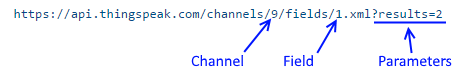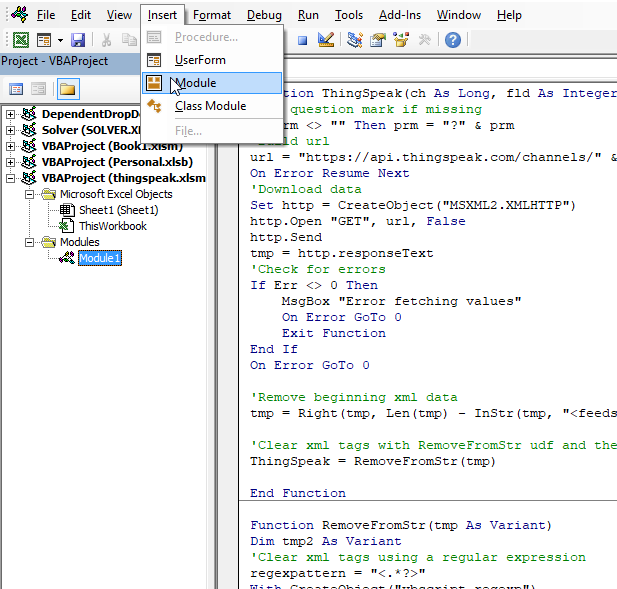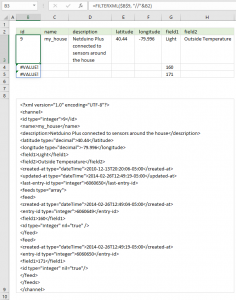Author: Oscar Cronquist Article last updated on July 06, 2021Picture above shows cheap chinese IoT modules: a temp sensor and an esp8266-01 (25 mm x 15 mm)

Thingspeak is a web service that allows you to upload and store data from IoT devices. IoT stands for Internet of Things.

Today I want to demonstrate a User Defined Function (UDF) that gets data from ThingSpeak to Excel so you can easily analyze and chart the data yourself, in Excel.

The way this works is that using a simple http address with your channel and field number and parameters, you can easily fetch values from your feed.## 2. XML example

The HTTP address returns XML data, something like this:## 3. VBA code

The following UDF lets you specify a channel, field, and some more parameters. The function then removes the XML tags and returns data to the worksheet.

Remember to enter this as an array formula, 3 columns wide.

```'Name function and define parameters
Function ThingSpeak(ch As Long, fld As Integer, prm As String)

'Add question mark if missing
If prm &lt;&gt; "" Then prm = "?" &amp; prm

'Build url
url = "https://api.thingspeak.com/channels/" &amp;ach &amp; "/fields/" &amp; fld &amp; ".xml" &amp; prm

'Enable error handling
On Error Resume Next

'Get data
Set http = CreateObject("MSXML2.XMLHTTP")
http.Open "GET", url, False
http.Send
tmp = http.responseText

'Check for errors
If Err &lt;&gt; 0 Then

'Show message box
MsgBox "Error fetching values"

'Disable error handling
On Error GoTo 0

'Stop
Exit Function
End If

'Disable error handling
On Error GoTo 0

'Remove beginning xml data
tmp = Right(tmp, Len(tmp) - InStr(tmp, "") + 1)

'Clear xml tags with RemoveFromStr udf and then return values to worksheet
ThingSpeak = RemoveFromStr(tmp)

End Function
```

## 4. Remove XML tags

```'Name User Defined Function and define parameters
Function RemoveFromStr(tmp As Variant)

'Dimension variables and declare data types
Dim tmp2 As Variant

'Clear xml tags using a regular expression
regexpattern = "&lt;.*?&amp;&gt;"
With CreateObject("vbscript.regexp")
.Global = True
.MultiLine = True
.IgnoreCase = False
.Pattern = regexpattern
Set Results = .Run(tmp)
End With

'Check if there are matching strings
If Results.Count &lt;&gt; 0 Then

'With ... End With statement
With Results

'For ... Next statement
'Iterate through matching strings
For d = 0 To .Count - 1

'Replace matching string with a comma
tmp = Replace(tmp, .Item(d), ",")
Next
End With
End If

'Remove spaces
tmp = Replace(tmp, " ", "")

'Remove newline
tmp = Replace(tmp, vbLf, "")

'Replace T and Z with space character
tmp = Replace(tmp, "T", " ")
tmp = Replace(tmp, "Z", " ")

'Combine multiple commas
For i = 1 To Len(tmp)
If Mid(tmp, i, 1) = "," And Mid(tmp, i, 1) = Mid(tmp, i + 1, 1) Then
Else
tmp1 = tmp1 &amp; Mid(tmp, i, 1)
End If
Next i

'Remove beginning and ending commas
If Left(tmp1, 1) = "," Then tmp1 = Right(tmp1, Len(tmp1) - 1)
If Right(tmp1, 1) = "," Then tmp1 = Left(tmp1, Len(tmp1) - 1)

'Split text into 1D array
tmp1 = Split(tmp1, ",")

'Count array
j = ((UBound(tmp1) - LBound(tmp1) + 1) / 3) - 1

'Build 2D array
ReDim tmp2(0 To j, 0 To 2)
ro = 0
co = 0
For i = LBound(tmp1) To UBound(tmp1)
tmp2(ro, co) = tmp1(i)
co = co + 1
If co = 3 Then
co = 0
ro = ro + 1
End If
Next i
'Return array to worksheet
RemoveFromStr = tmp2

End Function

```

## 5. Where do I put the VBA code?1. Open Visual Basic Editor. (shortcut keys Alt + F11).
2. Press with mouse on "Insert" on the top menu, a popup menu appears. See the above image.
3. Press with mouse on "Module" to insert a new module.
4. Copy VBA code.
5. Paste VBA code to code window, see image above.
Note, save your workbook with file extension *.xlsm (macro-enabled workbook) to attach the code.

## 6. Instructions - how to use the UDF#### 6.1 User Defined Function - Syntax

ThingSpeak(c, fld, prm)

c - channel
fld - field
prm - parameter

#### 6.2 How to enter the UDF as an array formula

1. Select cell range A1:C4.
2. Type =ThingSpeak(9,1,"results=4") in formula bar, see the image above.
3. Press and hold CTRL and SHIFT keys simultaneously.
4. Press Enter once.
5. Release all keys.
6. Excel automatically inserts curly brackets before and after the formula, see the formula bar in the image above.

## 7. Extract XML using a formula - FILTERXML (Excel 2013)The image above demonstrates how to extract data based on XML from ThingSpeak using the FILTERXML function. The column headers located in row 2 is used to extract the corresponding data from cell B9.

Formula in cell B3:

=FILTERXML(\$B\$9, "//"&B2)